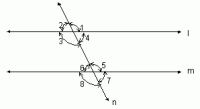Email us to get an instant 20% discount on highly effective K-12 Math & English kwizNET Programs!

#### Online Quiz (WorksheetABCD)

Questions Per Quiz = 2 4 6 8 10

### Geometry1.19 Angles Made by a Transversal With Two Parallel Lines - IIExample : In the above figure l//m; n is the transversal. If Đ1 = 110°, find the other angles ? Solution : Given that Đ1 = 110°. Đ1 =Đ5, since Đ1 and Đ5 are corresponding angles. Therefore Đ5 = 110° Đ5 = Đ3, since they are interior alternate angles. Therefore Đ3 = 110°. Đ3 = Đ 7, since they are corresponding angles. Therefore Đ7 = 110°. Đ4 + Đ5 = 180°, since Đ4 and Đ5 are the interior angles those are on the same side of the transversal. But Đ5 = 110° Therefore Đ4 = 180° - 110° = 70°. Đ4 = Đ6, since they are interior alternate angles. Therefore Đ6 = 70°. Đ2 = Đ6, since they are corresponding angles. Therefore Đ2 = 70°. Therefore Đ1 = 110° , Đ2 = 70°, Đ3 = 110°, Đ4 = 70°, Đ5 = 110°, Đ6 =70°, Đ7 = 110° and Đ8 = 70°. Directions:Answer the following questions. Also draw parallel lines with a transversal and show all the angles formed by them and write all the sets of congruent and supplementary angles.>
 Q 1: If angle 2 = 60 degrees, find angle 5.120 degrees130 degrees80 degrees Q 2: What is the sum of the measures of angle 1 and angle 8?180 degrees160 degrees130 degrees Q 3: What is the sum of the measures of angle 3 and angle 6?130 degrees90 degrees180 degrees Q 4: If angle 8 = 120 degrees, find angle 3.100 degrees120 degrees130 degrees Question 5: This question is available to subscribers only! Question 6: This question is available to subscribers only!